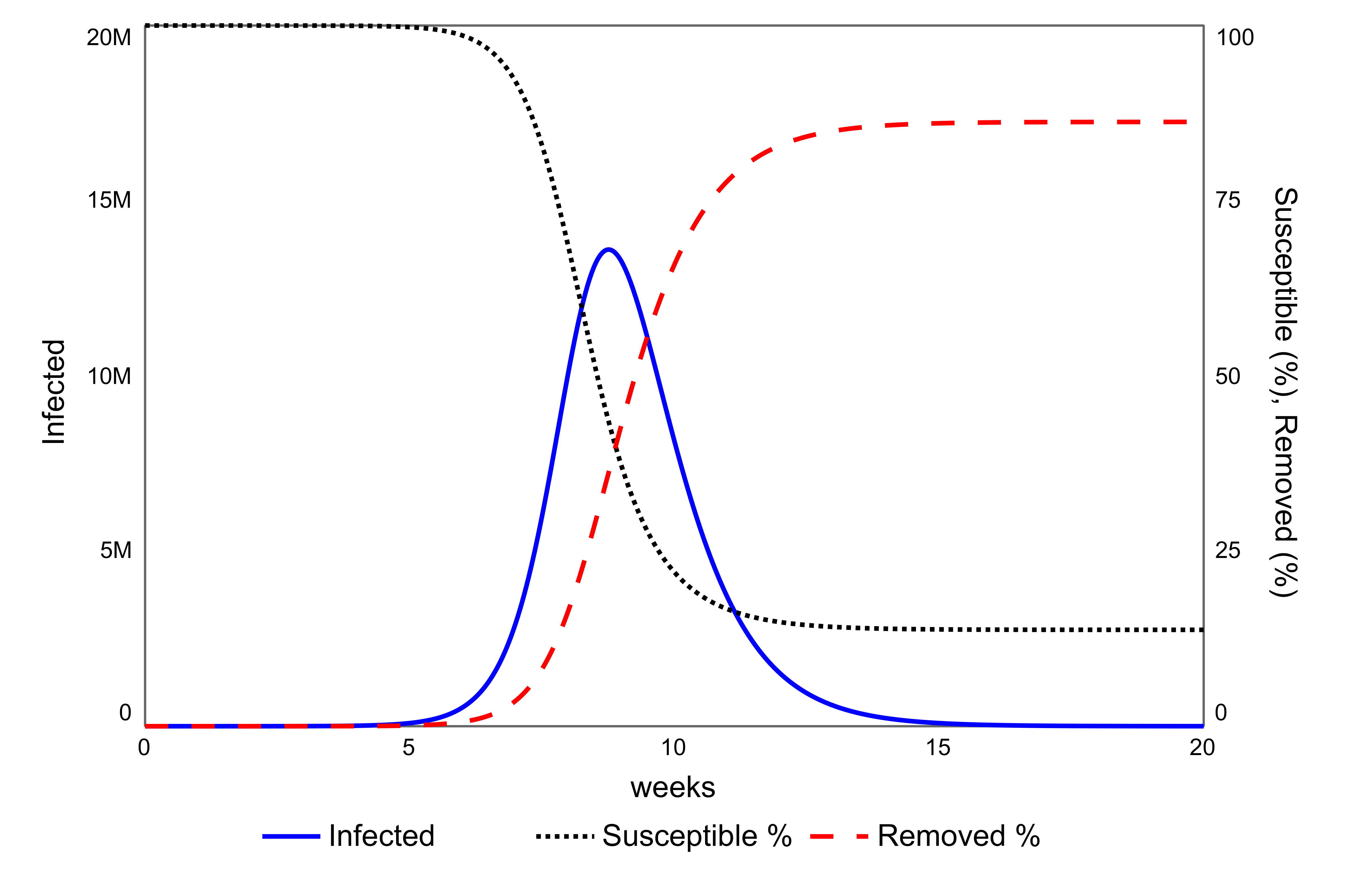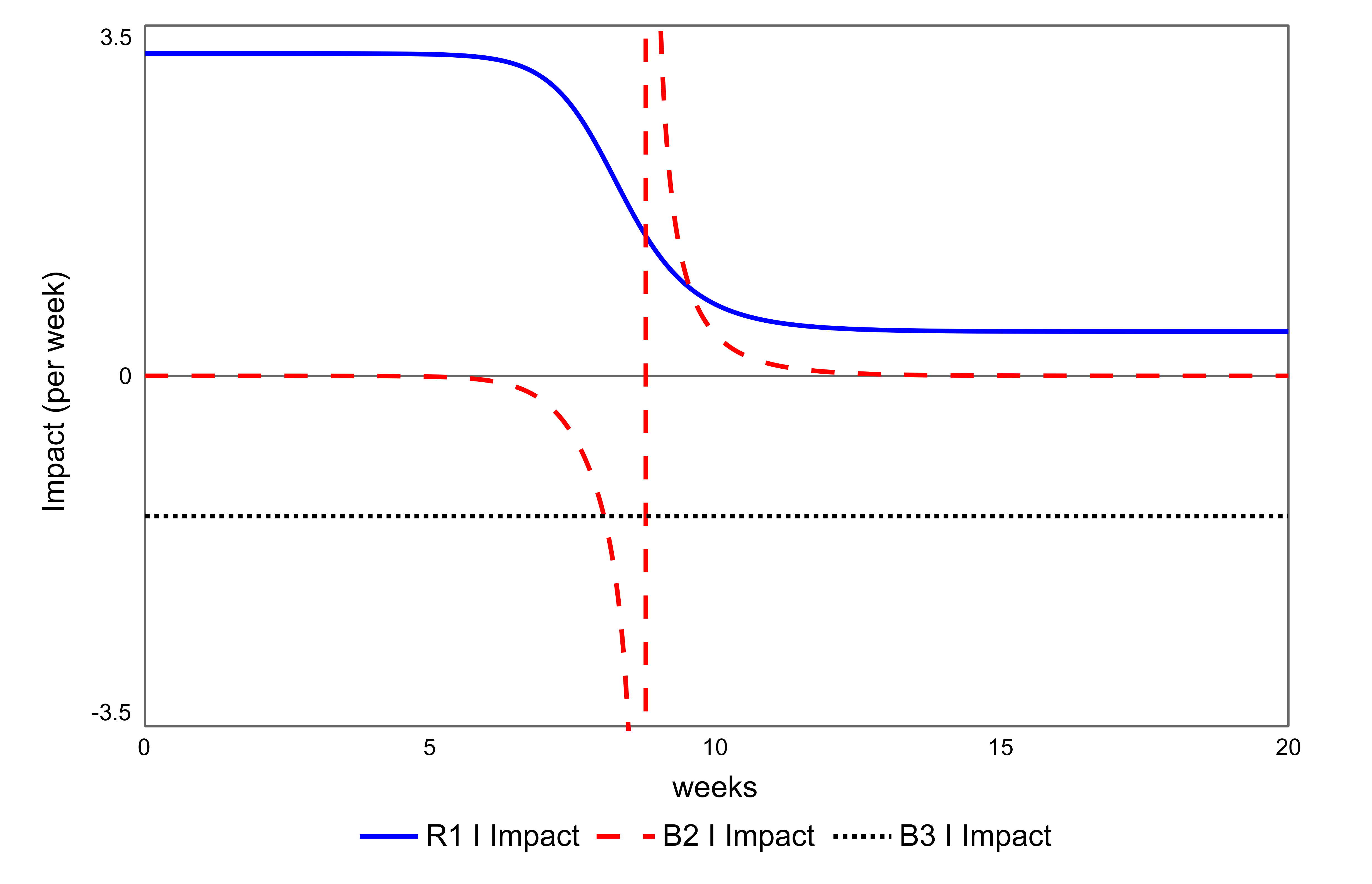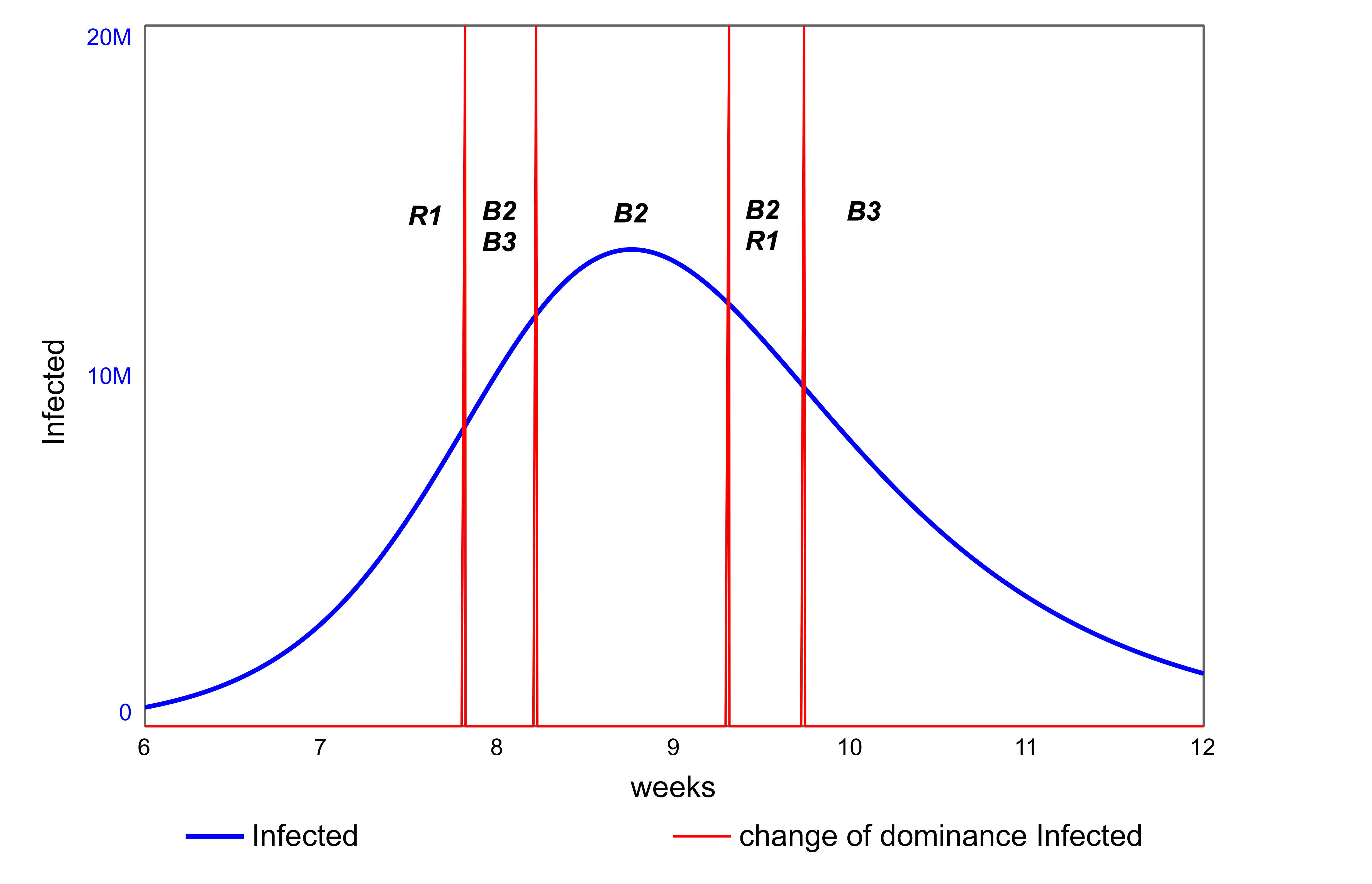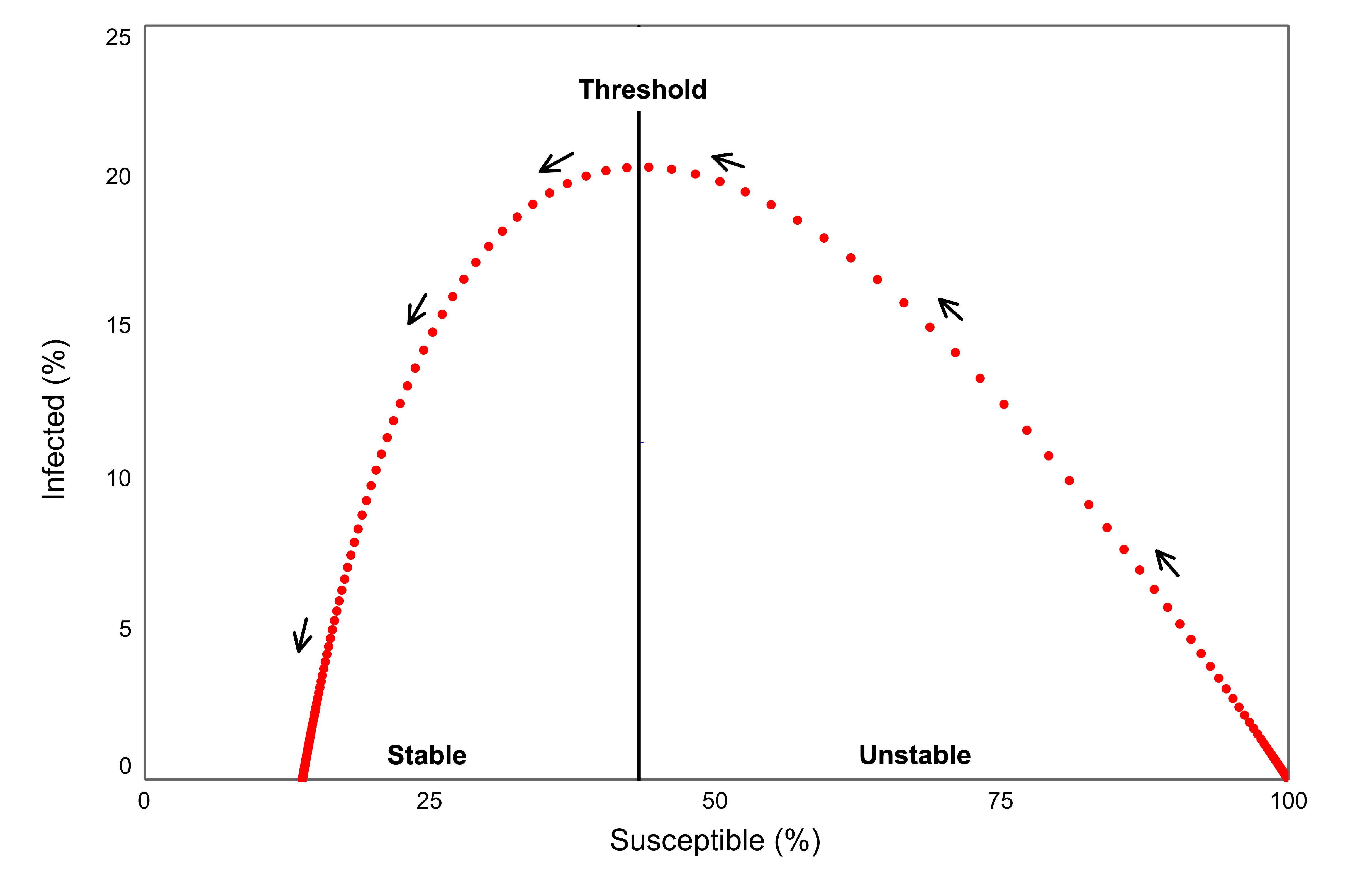# SIR Model

The SIR model describes the spread of a disease through a population. The model was originally proposed by Kermack and McKendrick in 1926 using differential equations, however it can also be presented in system dynamics form using stocks and flows. This modelling style will help clarify the feedback loops.

The population is split into three stocks: Susceptibles (S) – those who are able to catch the disease; Infected (I) – those infected with the disease and capable of passing it on to others; and Removed (R) – those cured of the disease and now immune to further infection, figure 1. The parameter duration infected is the average length of time a person is infectious. A critical factor in the rate of spread of a disease is the number of people one infected person infects with the disease during the period they are infectious. This is termed the reproductive ratio and denoted R0. If R0<1, then the number of infected decreases and the disease dies out. If R0>1, the number of infected increases and there is an epidemic. The larger R0, the larger the epidemic.

### Simulation

The model is simulated starting with a small number of infected people in a population of 67 million, figure 2. The growth of the infected starts very slowly – there are only a few thousand infected by the end of week 3. However, it soon accelerates, giving 13 million infected by week 9.Figure 2: Results of the SIR model. Infected on left axis, susceptible and removed as a percentage of the population on the right axis. R0 = 2.3, duration infected = 5 days, initial infected = 10.

The change in the infected stock is controlled by three feedback loops: R1 – a reinforcing loop controlling the uptake of infection; B2 – a balancing loop controlling the depletion of the susceptible population, affecting the infected stock through its inflow; and B3 – controlling the recovery of the infected. Using the Newtonian Interpretative Framework (Hayward & Roach, 2017, 2019), these loops can be thought of as forces that determine the acceleration of the number of infected :

• R1 is a driving force caused by the infected contacting susceptibles;
• B2 is a resistive force due to the depletion of susceptible numbers;
• B3 is another resistive or diffusive force caused by the loss of infected.

### Determining the Forces

The influence of these forces can be determined by expressing the stock-flow model as three causally connected differential equations, which preserves the network of connections in figure 1:

The indices 1-3 identify the causal path to the stock (variable) with the feedback loops, (R1, B2 and B3). The symbol τ represents the duration infected and N the number of people in the population.

The three forces are measured by the Loop Impact of their respective loops. Loop impact is determined by the ratio of the force’s acceleration to the rate of change of the variable (Hayward & Boswell, 2014). It is a measure of the curvature of the graph of the infected in units per week. The loop impacts are computed by pathway differentiation (Hayward & Roach, 2017, 2019):

The “bar” indicates the variable is a fraction of the population, e.g.

The three forces are plotted in figure 3.Figure 3. The three forces on the Infected stock in the SIR model, measured by the impact of the feedback loops. R0 = 2.3, duration infected = 5 days, initial infected = 10.

### Force Behaviour

In the first six weeks, R1, the force driving the acceleration of infected, is by far the largest of the three. Although the infected start with a small number and a low rate of change, the driving force is large.

By contrast, the resistive force B2 is negligible initially as the susceptible numbers are so far above the epidemic threshold. For the infected to grow:

where ρ is the epidemic threshold, given by the inverse of the reproductive ratio. For R0 = 2.3, the number infected will increase as long as more than 43% of the population is susceptible.

The resistive force from B3 is constant as the feedback loop is linear, but it is too small to oppose R1.

As the number infected starts to accelerate, the impact of the driving force R1 drops due to the pool of susceptibles shrinking. Correspondingly, the resistive force of the susceptibles B2 increases. Some of the infecteds’ contacts are with those who are now in the removed population, who are immune, which weakens R1 and strengthens B2. A point is reached at which the combination of the two resistive forces exceeds that of R1 and the increase in the infected slows until its numbers peak. At this point, the infected numbers decrease with B2 accelerating the decline. Both R1 and B2 weaken, leaving the resistive force B3 dominant, slowing down the decline of the infected until it reaches zero.

### Force Transitions

The transitions between forces is indicated in figure 4:Figure 4: Transitions in force/loop dominance on the infected in the SIR model. R0 = 2.3, duration infected = 5 days, initial infected = 10.

When the epidemic ends, some susceptibles remain, having fallen to a value below the threshold, figure 5. However, with intervention, it is possible to stop the epidemic with fewer people infected, leaving a larger number of susceptibles at the end, at a value up to, but not exceeding, the threshold, (of 43% in this case). Interventions correspond to another form of resistive force. Figure 3 indicates that such interventions would be more successful if applied later in the accelerating phase when the driving force R1 has come weaker and the natural resistance of the shrinking susceptible pool, B2, is strong enough to assist the intervention.Figure 5: Phase plot of the SIR model. The arrows indicate the direction of time. R0 = 2.3, duration infected = 5 days, initial infected = 10.

An epidemic ends when the number of infected becomes zero, the susceptible axis in figure 5. Thus, the whole of the susceptible axis is in equilibrium. However, only the region below the threshold is stable. The region above the threshold is unstable and, therefore, cannot be reached without continual interventions, such as quarantine, which would affect the structure of the model, figure 1.